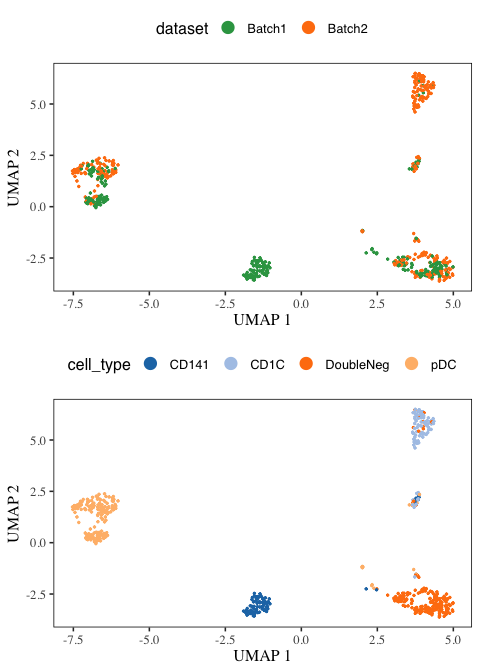# Introduction

SCIBER is a simple method that outputs the batch-effect corrected expression data in the original space/dimension. These expression data of individual genes can be directly used for all follow-up analyses. SCIBER has four steps; each step has a clear biological meaning, and the algorithms used for them are k-means clustering, t-test, Fisher’s exact test, and linear regression, respectively, all of which are easily comprehensible.

# Installation

Install SCIBER with standard commands,

install.packages('SCIBER')

or install the development version of SCIBER with the following commands.

# install.packages("devtools")
devtools::install_github("RavenGan/SCIBER")

Once SCIBER is installed, load it.

library(SCIBER)

# Removing batch effects in Human dendritic cells.

We downloaded two batches of Human dendritic cell data from this paper

Villani, A. C., Satija, R., Reynolds, G., Sarkizova, S., Shekhar, K., Fletcher, J., … & Hacohen, N. (2017). Single-cell RNA-seq reveals new types of human blood dendritic cells, monocytes, and progenitors. Science, 356(6335), eaah4573.

We library normalized the cells, log transformed the counts, and selected top 500 highly variable genes for each batch. We pooled all the genes and use them as the genes for both batches. The pre-processed data are available as part of this package.

data("HumanDC")
exp <- HumanDC[["exp"]]
meta <- HumanDC[["metadata"]]

We first specify the parameter we want to use in SCIBER. We set omega = 0.5 which is also the default setting in SCIBER. Setting ref_index = 1 indicates the first bacth is treated as the reference batch while the second is the query batch. By using n_core = 1, we only use 1 core to run SCIBER.

omega <- c()
omega[] <- 0.5

ref_index <- 1
n_core <- 1

Let’s run SCIBER to remove the batch effects.

res <- SCIBER(input_batches = exp, ref_index = ref_index,
batches_meta_data = meta, omega = omega, n_core = n_core)
#>  "The available number of cores is 10. SCIBER uses 1 to perform batch effect removal."

The output of SCIBER is a list of batches, which is the same as the input exp. The order of batches in res is the same as that of exp.

Next, we combine the output batches, do PCA and UMAP before plotting them.

library(stats)
library(Matrix)
library(uwot)

do_PCA <- function(dat, PCs){
dat_pca_embeddings <- prcomp(t(as.matrix(dat)), scale. = F)
dat_pca_embeddings <- dat_pca_embeddings$x dat_pca_embeddings <- dat_pca_embeddings[, 1:as.numeric(PCs)] return(dat_pca_embeddings) } do_umap <- function(V) { umap( X = V, n_threads = 6, n_neighbors = 30L, n_components = 2L, metric = 'cosine', n_epochs = NULL, learning_rate = 1.0, min_dist = 0.3, spread = 1.0, set_op_mix_ratio = 1.0, local_connectivity = 1L, repulsion_strength = 1, negative_sample_rate = 1, a = NULL, b = NULL, fast_sgd = FALSE, verbose = FALSE ) } meta_data <- rbind(meta[], meta[]) rownames(meta_data) <- meta_data$cell_id

projected_dat <- cbind(res[], res[])

all(rownames(meta_data) == colnames(projected_dat))
#>  TRUE

SCIBER_pca <- do_PCA(projected_dat, PCs = 20)
SCIBER_umap <- do_umap(SCIBER_pca)

Then, we load necessary packages and function for plots.

library(dplyr)
#>
#> Attaching package: 'dplyr'
#> The following objects are masked from 'package:stats':
#>
#>     filter, lag
#> The following objects are masked from 'package:base':
#>
#>     intersect, setdiff, setequal, union
library(ggplot2)
library(ggthemes)
library(cowplot)
#>
#> Attaching package: 'cowplot'
#> The following object is masked from 'package:ggthemes':
#>
#>     theme_map

obtain_plot <- function(
umap_use,
meta_data,
label_name,
palette_use = tableau_color_pal()(10),
pt_size = 4, point_size = 0.5, pt_shape = '.',
base_size = 12,
do_points = TRUE,
do_density = FALSE,
legend_position = "top"
){
plt_df <- umap_use %>% data.frame() %>% cbind(meta_data) %>%
sample_frac(1L)
plt <- plt_df %>%
ggplot(aes_string("X1", "X2", col = label_name,fill = label_name)) +
theme_tufte(base_size = base_size) +
theme(panel.background = element_rect(fill = NA, color = "black")) +
guides(color = guide_legend(override.aes = list(stroke = 1,
alpha = 1, shape = 16, size = 4)),
alpha = FALSE) +
scale_color_manual(values = palette_use, guide = "none") +
scale_fill_manual(values = palette_use, guide = "none") +
theme(plot.title = element_text(hjust = 0.5, family = "sans"),
legend.text = element_text(family = "sans"),
legend.title = element_text(family = "sans"),
legend.position= as.character(legend_position)) +
labs(x = "UMAP 1", y = "UMAP 2")

if (do_points)
plt <- plt + geom_point(shape = pt_shape, size = point_size)
if (do_density)
plt <- plt + geom_density_2d()

return(plt)
}

Choose colors for cell types and batches.

colors_cell <- tableau_color_pal("Classic 20",
direction = 1)(length(unique(meta_data$cell_type))) colors_batch <- tableau_color_pal("Classic Green-Orange 6", direction = 1)(length(unique(meta_data$dataset)))

Let’s see the umap plots!

SCIBER_plt1 <- obtain_plot(SCIBER_umap, meta_data, "dataset", palette_use = colors_batch,
pt_shape = 19, pt_size = .4, legend_position = "top")
#> Warning: guides(<scale> = FALSE) is deprecated. Please use guides(<scale> =
SCIBER_plt2 <- obtain_plot(SCIBER_umap, meta_data, "cell_type", palette_use = colors_cell,
pt_shape = 19, pt_size = .4, legend_position = "top")
#> Warning: guides(<scale> = FALSE) is deprecated. Please use guides(<scale> =

plot_grid(SCIBER_plt1, SCIBER_plt2, nrow = 2)# Session Info

sessionInfo()
#> R version 4.1.2 (2021-11-01)
#> Platform: x86_64-apple-darwin17.0 (64-bit)
#> Running under: macOS Big Sur 10.16
#>
#> Matrix products: default
#> BLAS:   /Library/Frameworks/R.framework/Versions/4.1/Resources/lib/libRblas.0.dylib
#> LAPACK: /Library/Frameworks/R.framework/Versions/4.1/Resources/lib/libRlapack.dylib
#>
#> locale:
#>  C/en_US.UTF-8/en_US.UTF-8/C/en_US.UTF-8/en_US.UTF-8
#>
#> attached base packages:
#>  stats     graphics  grDevices utils     datasets  methods   base
#>
#> other attached packages:
#>  cowplot_1.1.1  ggthemes_4.2.4 ggplot2_3.3.6  dplyr_1.0.9    uwot_0.1.11
#>  Matrix_1.4-1   SCIBER_0.1.5
#>
#> loaded via a namespace (and not attached):
#>   Rcpp_1.0.8.3     RSpectra_0.16-1  highr_0.9        pillar_1.7.0
#>   bslib_0.3.1      compiler_4.1.2   jquerylib_0.1.4  tools_4.1.2
#>   digest_0.6.29    gtable_0.3.0     jsonlite_1.8.0   evaluate_0.15
#>  lifecycle_1.0.1  tibble_3.1.7     lattice_0.20-45  pkgconfig_2.0.3
#>  rlang_1.0.4      cli_3.3.0        DBI_1.1.3        rstudioapi_0.13
#>  yaml_2.3.5       parallel_4.1.2   xfun_0.31        fastmap_1.1.0
#>  withr_2.5.0      stringr_1.4.0    knitr_1.39       generics_0.1.3
#>  vctrs_0.4.1      sass_0.4.1       grid_4.1.2       tidyselect_1.1.2
#>  glue_1.6.2       R6_2.5.1         RcppAnnoy_0.0.19 fansi_1.0.3
#>  rmarkdown_2.14   farver_2.1.0     purrr_0.3.4      magrittr_2.0.3
#>  scales_1.2.0     codetools_0.2-18 ellipsis_0.3.2   htmltools_0.5.2
#>  assertthat_0.2.1 colorspace_2.0-3 labeling_0.4.2   utf8_1.2.2
#>  stringi_1.7.6    munsell_0.5.0    crayon_1.5.1